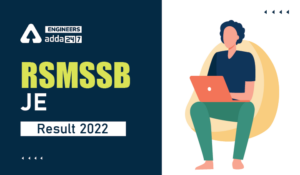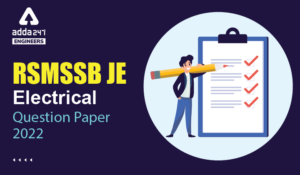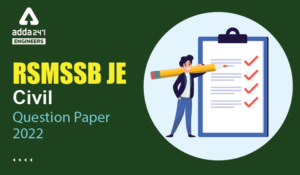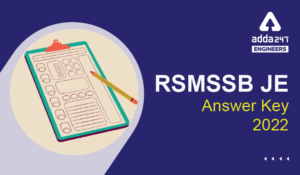Engineering Jobs   »   Civil Engineering quizs   »   RSMSSB-JE

# RSMSSB-JE’21 CE: Daily Practices Quiz. 09-Sep-2021

As RSMSSB JE exam date 2021 is announced which is going to be held on 12th September 2021. As RSMSSB JE exam date 2021 is nearby so boost your preparation by attempt this Civil Engineering miscellaneous quiz for RSMSSB JE recruitment 2021.

Quiz: Civil Engineering
Exam: RSMSSB-JEn
Topic: Miscellaneous

Each question carries 1 mark
Negative marking: 1/3 mark
Time: 8 Minutes

Q1. Which of the following relation is/are used in deriving the relation between the flow rate and the pressure difference between the straight section and the throat of a venturi-meter that has no losses?
(a) ideal gas law
(b) Bernoulli and continuity equation
(c) Relation between friction factor and Reynolds number and Bernoulli equation
(d) Bernoulli equation

Q2. M-30 is considered as _________ type of concrete.
(a) Ordinary
(b) Standard
(c) High strength
(d) All of the above

Q3. If specific yield of particular sand is 30% and its porosity is 50%, then the specific retention of the sand will be
(a) 80%
(b) 55%
(c) 40%
(d) 20%

Q4. The ruling gradient for plain and rolling terrain is
(a) 3.3%
(b) 5%
(c) 6%
(d) 4 %

Q5. Estimate the quantity of plastering (two faces) for 4 m long, 3m high and 30 cm thick wall.
(a) 72 cum
(b) 2.4 sqm
(c) 24 sqm
(d) 36 cum

Q6. Void ratio of an undisturbed sample of soil is 0.6. the values of maximum and minimum possible void ratio are found as 0.8 and 0.4, respectively. The relative density in percentage, for this soil sample will be
(a) 25
(b) 50
(c) 75
(d) 90

Solutions

S1. Ans.(b)
Sol. For derive the relation between the flow rate and the pressure difference between the throat and straight section of a venturi meter we need continuity equation and Bernoulli’s equation both.

S2. Ans.(b)
Sol.

 Concrete Grade Type of concrete M10 – M20 Ordinary M25 – M55 Standard M60 – M80 High strength

S3. Ans.(d)
Sol. Given,
Specific yield (S_y) = 30%
Porosity (n) = 50%
Specific retention (SR) =?
S_y+S_R=n
30%+S_R= 50%
S_R=20%

S4. Ans.(a)
Sol. The ruling gradient for plain and rolling terrain is 3.3% or 1 in 30 while for mountainous terrain it is 5% or 1 in 20.

S5. Ans.(c)
Sol. Given, Length of wall (L) = 4m.
Height of wall (H) = 3 m.
Area of wall = L × H
= 4 × 3 = 12 m^2
Quantity of plaster (two faces) = 2 × 12
= 24 m^2

S6. Ans.(b)
Sol. e_max=0.8
e_min=0.4
e=0.6
Relative density = (e_(max )-e)/(e_max-e_min )×100
(0.8-0.6)/(0.8-0.4)×100
0.2/0.4×100
=50%

Sharing is caring!

Thank You, Your details have been submitted we will get back to you.

### TOPICS:

•RSMSSB JE 2022 DV Schedule, Download RSM...
•RSMSSB JE Result 2022, OUT-Direct Link t...
•RSMSSB Computer Instructor Exam date 202...
•RSMSSB JE Electrical Question Paper 2022...
•RSMSSB JE Civil Question Paper 2022, Che...
•RSMSSB JE Answer Key 2022, Download RSMS...